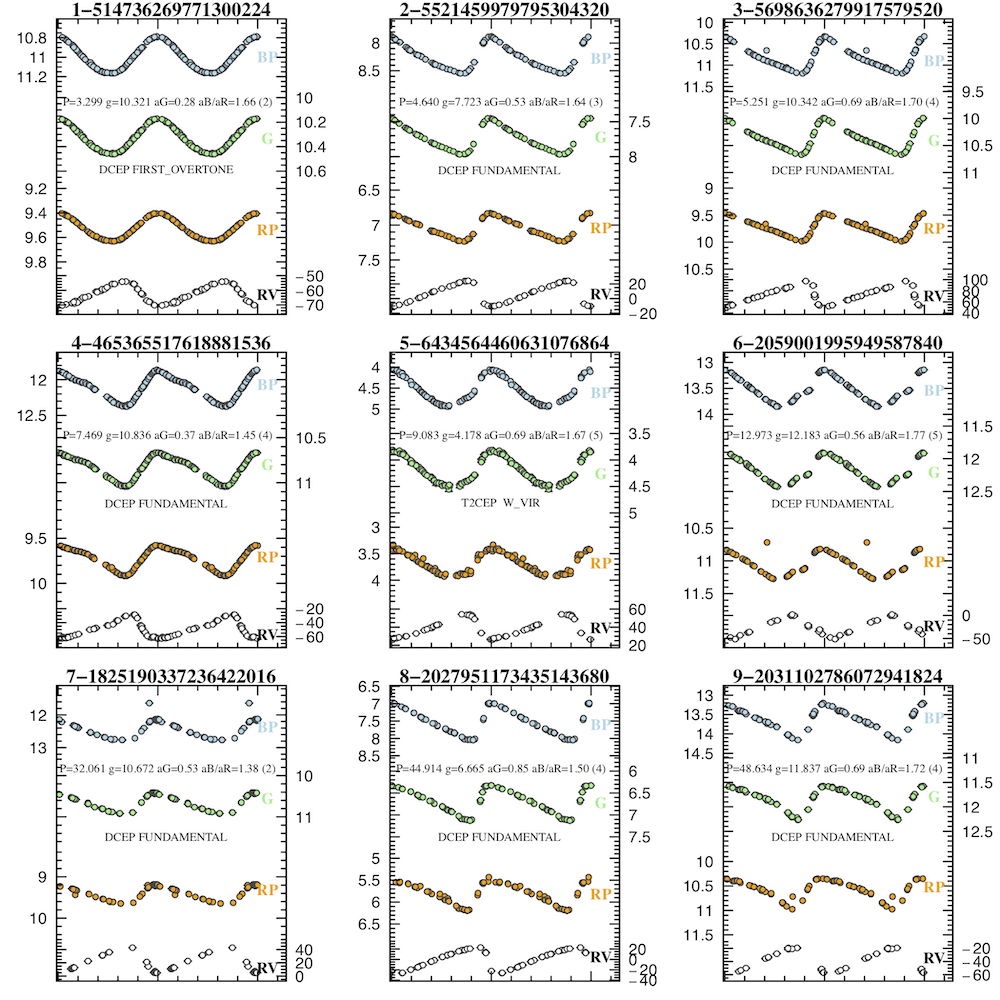# 10.5.2 Properties of the input data

Input data consisted of time-series in the $G$, $G_{\rm BP}$, $G_{\rm RP}$ bands (when $G_{\rm BP}$ and $G_{\rm RP}$ were available), and radial velocities for a sub-sample of Cepheids. Examples of light and radial velocity curves are shown in Figure 10.8.Figure 10.8: Examples of G, GBP, GRP light curves (upper three curves in each panel) and radial velocity curve (lower curve) for confirmed first overtone (1O) and fundamental mode (F) Classical Cepheids, and one W Virginis (type II Cepheid) stars. Units on the Y axis are mag for the light curves and km s-1 for the radial velocity curves. The data are folded according to the periods derived by the SOS Cep&RRL pipeline and plotted over two cycles. A few outliers not removed by the outlier removal operators are still present in a couple of cases, however they do not affect the light curve models and mean G, GBP, GRP magnitudes computed by the SOS Cep&RRL pipeline.

The sources analysed by the package were selected according to the following criteria:

• sources classified as candidate Cepheid variables from the Classifiers;

• a minimum number of 12 $G$-FoV transits, after applying the general outlier removal
MultibandOutlierRemovalOperator (Section 10.2.3), as well as the
RemoveOutliersFaintAndBrightOperator outlier removal operator, whose parameters were fine tuned to discard residual outliers in the Cepheid light curves;

• a minimum number of five transits for the publication of the RV time-series and of eight transits for the modelling of the folded RV curve;

• a peak-to-peak amplitude $>$0.1 mag in the $G$-band;

• search of a second periodicity only for sources with a minimum number of more than 40 $G$-FoV transits and with scatter with respect to the modelled $G$-band light curve larger than 0.025 mag.